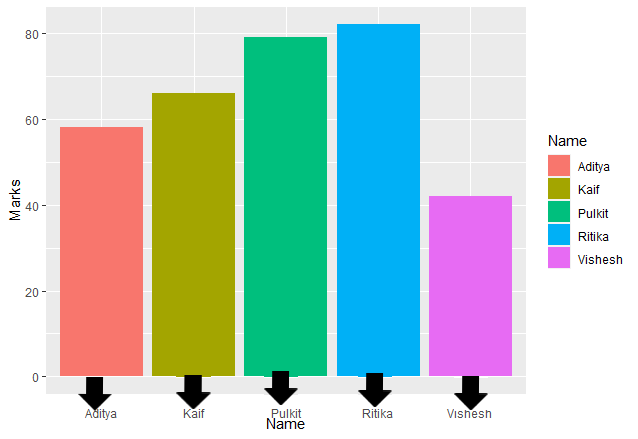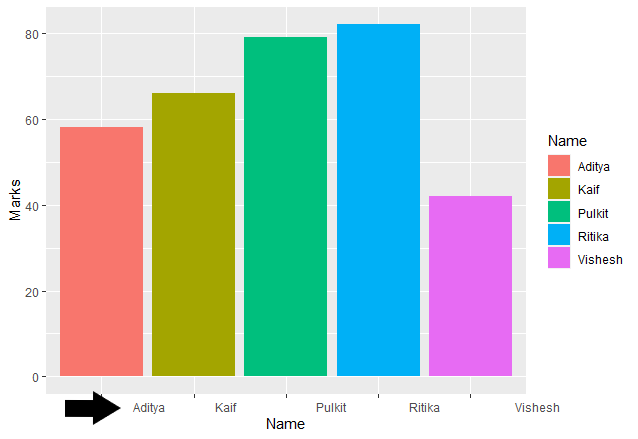# How to adjust Space Between ggplot2 Axis Labels and Plot Area in R ?

• Last Updated : 21 Apr, 2021

While plotting the graphs we can make different changes in order to make them much more appealing and clear to the observer. One of the ways is to adjust the spacing between the labels and plot area. In this article, we will study how to adjust space between ggplot2 Axis Labels and plot area in R Programming Language.

To add customizations to our plot we can use the theme() function.

### Method 1: Adjusting vertical spaces

To adjust the vertical spacing we use vjust in the element_text function to vertically adjust the plotting

Example

## R

 `rm``(list=``ls``())`` ` `data <- ``data.frame``(name = ``c``(``"Aditya"``, ``"Ritika"``, ``"Pulkit"``,``                            ``"Vishesh"``, ``"Kaif"``), ``                    ` `                   ``marks = ``c``(58, 82, 79, 42, 66))           ``                              ` `library``(``"ggplot2"``)   `` ` `ggp <- ``ggplot``(data, ``aes``(name, marks, fill = name)) +            ``  ``geom_bar``(stat = ``"identity"``)`` ` `ggp + ``theme``(axis.text.x = ``element_text``(vjust = -12))       `

Output:### Method 2: Adjust horizontal spacing

We can adjust the horizontal spacing in the similar way using hjust in element_text

Example

## R

 `rm``(list=``ls``())`` ` `data <- ``data.frame``(Name = ``c``(``"Aditya"``, ``"Ritika"``, ``"Pulkit"``,``                            ``"Vishesh"``, ``"Kaif"``), ``                    ` `                   ``Marks = ``c``(58, 82, 79, 42, 66))           `` ` `library``(``"ggplot2"``)                                          `` ` `ggp <- ``ggplot``(data, ``aes``(Name, Marks, fill = Name)) +            ``  ``geom_bar``(stat = ``"identity"``)                        `` ` `ggp + ``theme``(axis.text.x = ``element_text``(hjust = -1))   `

Output: